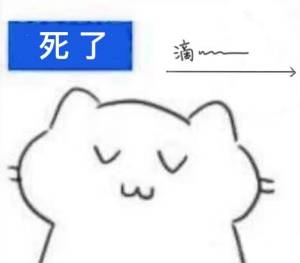# 抱歉，您的浏览器无法访问本站

## 题解

### D - Cut

• 我们可以倒着维护每一个质数的下一个位置，这样就不用对每个质数二分查找了
• 倒着转移的时候也考虑后一个位置的备选位置，这样就不用考虑到备选区间之间的冲突了

### E - Baby Ehab Plays with Permutations

• 如果 $$p_n = n$$，那么可以忽略这个位置；将原排列看作长度为 $$n - 1$$
• 否则，将 $$p_n$$$$p_{p_n}$$ 交换位置；此时至少可以使得 $$p_n$$ 复位，继续递归，但是操作次数 $$+1$$

• $$j = 0$$：此时，只有初始复位的排列一种情况；因此总是为 $$1$$
• 现在，我们考虑通过 $$F_{n - 1}$$$$F_n$$ 转移；即每次将 $$n$$ 放入长度为 $$n - 1$$ 的排列中：
• 如果新放入的 $$n$$ 不进行交换，那么对答案没有贡献：可以直接转移
• 如果和前面的 $$n - 1$$ 个位置中的一个进行交换：那么贡献 $$1$$ 次次数，有 $$n - 1$$ 种转移方法
• 综上所述，可以得到递推公式 $$F_{n, j} = F_{n - 1, j} + (n - 1) \cdot F_{n - 1, j - 1}$$

#### 阳间做法

• 现在，我们定义 $$G_{n, j}$$$$F_{n, j}$$ 类似，但是每一个位置都是错排的情况数量
• 然后，我们在 $$F_{n, j}$$ 中选择一个位置固定，然后剩下的位置的任何排序就都符合要求，需要排除
• 但是这样的话，就会导致两个位置固定的情况被多排除了一次，需要重新包含
• ……

#### 阴间做法

• 考虑到 $$F_{n, 0} = 1$$，除了递推的转移引入之外，都是通过 $$n - 1$$ 的形式引入的；
• 什么情况下会引入 $$n - 1$$？当新加入的 $$n$$ 不放在 $$[n]$$ 而是参与了与前面的位置交换的场合下；
• 引入时产生了什么副作用？因为进行了交换，所以奉献了一次交换次数，$$j = j + 1$$
• 所以，我们可以将 $$F_{n, j}$$ 看作从“下标”集合 $$[0, n - 1]$$ 中选出一个大小 $$j$$ 的子集求积后求和的结果；

• 一个很显然的想法，就是我们从 $$[0, n - 1]$$ 中选择 $$l$$ 个，从 $$[n, 2n - 1]$$ 中选出剩下的，组成上面所提到的从集合 $$[0, 2n-1]$$ 中选出的大小为 $$j$$ 子集；形式化地说就是将两部分的结果乘起来

• 前半部分的答案很显然是 $$F_{n, l}$$，而后半部分的答案我们没有维护；但是我们可以把它看作从 $$[0, n - 1]$$ 中选择了 $$j - l$$ 个，然后对于每一个都加上了 $$n$$，也就是下面这样： $F'_{n, j'} = \sum_{s\subset [n, 2n-1] \and |s| = j'} \ \prod_{i = 0}^{j'}s_{i} = \sum_{s\subset [0, n-1] \and |s| = j'} \ \prod_{i = 0}^{j'}(s_{i} + n)$ 那么这个式子展开是什么样的呢？因为这个连乘长得非常像二项式展开但是不是，残念（，所以我们可以想象一下它展开后的样子： $F'_{n, j'} = \sum_{l = 0}^{j'} \mathbf{C}_{n - l}^{j'-l} \times n^{j'-l} \times \sum_{s\subset [0, n-1] \and |s| = l} \prod_{i = 0}^{l}s_i$ 上面的式子中，有 $$\mathbf{C}_{n - l}^{j' - l} = \mathbf{C}_n^{j'} \times \mathbf{C}_{j'}^l$$；首先是选出坐标的组合数，然后乘上“二项式系数”。

• 然后，观察上面的式子的后半部分，我们会惊喜地发现它就是 $$F_{n, l}$$，是我们已经维护的东西！

## 后记JVS2TR2_F28UCRC3K_YG_3B.jpg